## Model Discretizer

### What Is the Model Discretizer?

Model Discretizer selectively replaces continuous Simulink® blocks with discrete equivalents. Discretization is a critical step in digital controller design and for hardware in-the-loop simulations.

You can use the Model Discretizer to:

• Identify a model's continuous blocks

• Change a block's parameters from continuous to discrete

• Apply discretization settings to all continuous blocks in the model or selected blocks

• Create variant subsystems that contain multiple discretization candidates along with the original continuous block(s)

• Switch among the different discretization candidates and evaluate the resulting model simulations

The Model Discretizer does not support masked subsystems.

### Requirements

To use Model Discretizer

• You must have a Control System Toolbox™ license, Version 5.2 or later.

• You must convert configurable subsystem blocks in existing models to Variant Subsystem. For more information, see Convert Configurable Subsystem to Variant Subsystem.

### Discretize a Model with the Model Discretizer

To discretize a model:

The `f14` model shows the steps in discretizing a model.#### Start Model Discretizer

To open the tool, in the Simulink Editor, on the Apps tab, under Apps, under Control Systems, click Model Discretizer.Alternatively, you can open Model Discretizer from the MATLAB® Command Window using the `slmdldiscui` function.

The following command opens the Simulink Model Discretizer window with the `f14` model:

```slmdldiscui('f14') ```

To open a new model or library from Model Discretizer, select File > Load model.

#### Specify the Transform Method

The transform method specifies the type of algorithms used in the discretization. For more information on the different transform methods, see the Control System Toolbox.

The Transform method list contains the following options:

Transform MethodDescription

`Zero-order hold`

Zero-order hold on the inputs.

`First-order hold`

Linear interpolation of inputs.

`Tustin`

Bilinear (Tustin) approximation.

```Tustin with prewarping```

Tustin approximation with frequency prewarping.

`Matched pole-zero`

Matched pole-zero method (for SISO systems only).

#### Specify the Sample Time

Enter the sample time in the Sample time field. For the Model Discretizer, this value must be numeric.

You can specify an offset time by entering a two-element vector for discrete blocks or variant subsystems. The first element is the sample time and the second element is the offset time. For example, an entry of [1.0 0.1] would specify a 1.0 second sample time with a 0.1 second offset. If no offset is specified, the default is zero.

You can enter workspace variables when discretizing blocks in the s-domain. See Discrete blocks (Enter parameters in s-domain).

#### Specify the Discretization Method

Specify the discretization method in the Replace current selection with field. The options are

Discretization MethodDescription

Discrete blocks (Enter parameters in s-domain)

Creates a discrete block whose parameters are retained from the corresponding continuous block.

Creates a discrete block whose parameters are “hard-coded“ values placed directly into the block's dialog.

Create multiple discretization candidates using s-domain values for the current selection.

Create multiple discretization candidates in z-domain for the current selection.

Discrete blocks (Enter parameters in s-domain).  Creates a discrete block whose parameters are retained from the corresponding continuous block. The sample time and the discretization parameters are also on the block's parameter dialog box.

The block is implemented as a masked discrete block that uses `c2d` (Control System Toolbox) to transform the continuous parameters to discrete parameters in the mask initialization code.

These blocks have the unique capability of reverting to continuous behavior if the sample time is changed to zero. Entering the sample time as a workspace variable (`'Ts'`, for example) allows for easy changeover from continuous to discrete and back again. See Specify the Sample Time.

Note

If you generated code from a model, parameters are not tunable when Default parameter behavior is set to `Inlined` in the model's Configuration Parameters dialog box.

The following figure shows a continuous Transfer Function block next to a Transfer Function block that has been discretized in the s-domain with the Tustin transform method. The block parameters dialog box for each block appears below the block.Discrete blocks (Enter parameters in z-domain).  Creates a discrete block whose parameters are “hard-coded” values placed directly into the block's dialog box. Model Discretizer uses the `c2d` (Control System Toolbox) function to obtain the discretized parameters, if needed.

For more help on the `c2d` (Control System Toolbox) function, type the following in the Command Window:

```help c2d ```

The following figure shows a continuous Transfer Function block next to a Transfer Function block that has been discretized in the z-domain. The block parameters dialog box for each block appears below the block.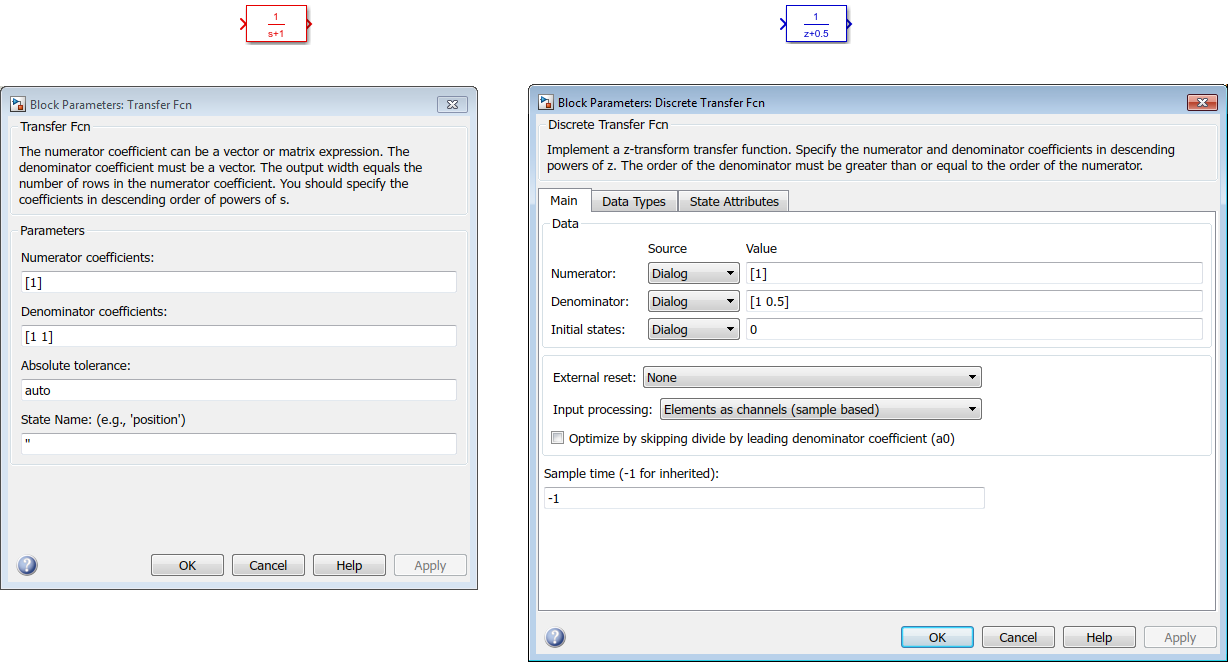Note

If you want to recover exactly the original continuous parameter values after the Model Discretization session, you should enter parameters in the s-domain.

Variant subsystem (Enter parameters in s-domain).  Create multiple discretization candidates using s-domain values for the current selection. Creates a discrete block whose parameters are retained from the corresponding continuous block.​

The Location for block in Variant Subsystem field becomes active when this option is selected. This option allows you to either create a new variant subsystem.

Variant subsystem (Enter parameters in z-domain).  Create multiple discretization candidates in z-domain for the current selection. Creates a discrete block whose parameters are “hard-coded“ values placed directly into the block's dialog.​

The Location for block in Variant Subsystem field becomes active when this option is selected. This option allows you to either create a new variant subsystem.

#### Discretize the Blocks

To discretize blocks that are linked to a library, you must either discretize the blocks in the library itself or disable the library links in the model window.

You can open the library from Model Discretizer by selecting Load model from the File menu.

There are two methods for discretizing blocks:

Select Blocks and Discretize

1. Select a block or blocks in the Model Discretizer tree view pane.

To choose multiple blocks, press and hold the button on the keyboard while selecting the blocks.

Note

You must select blocks from the Model Discretizer tree view. Clicking blocks in the editor does not select them for discretization.

2. Select Discretize current block from the Discretize menu if a single block is selected or select Discretize selected blocks from the Discretize menu if multiple blocks are selected.

You can also discretize the current block by clicking the button, shown below.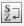Store the Discretization Settings and Apply Them to Selected Blocks in the Model

1. Enter the discretization settings for the current block.

2. Click Store Settings.

This adds the current block with its discretization settings to the group of preset blocks.

3. Repeat steps 1 and 2, as necessary.

4. Select Discretize preset blocks from the Discretize menu.

#### Deleting a Discretization Candidate from a Variant Subsystem

You can delete a discretization candidate from a variant subsystem by selecting it in the Location for block in Variant Subsystem field and clicking the button.

#### Undoing a Discretization

To undo a discretization, click the button.

Alternatively, you can select Undo discretization from the Discretize menu.

This operation undoes discretizations in the current selection and its children. For example, performing the undo operation on a subsystem will remove discretization from all blocks in all levels of the subsystem's hierarchy.

### View the Discretized Model

Model Discretizer displays the model in a hierarchical tree view.

#### Viewing Discretized Blocks

The block's icon in the tree view becomes highlighted with a “z” when the block has been discretized.

The following figure shows that the Aircraft Dynamics Model subsystem has been discretized into a variant subsystem with two discretization candidates.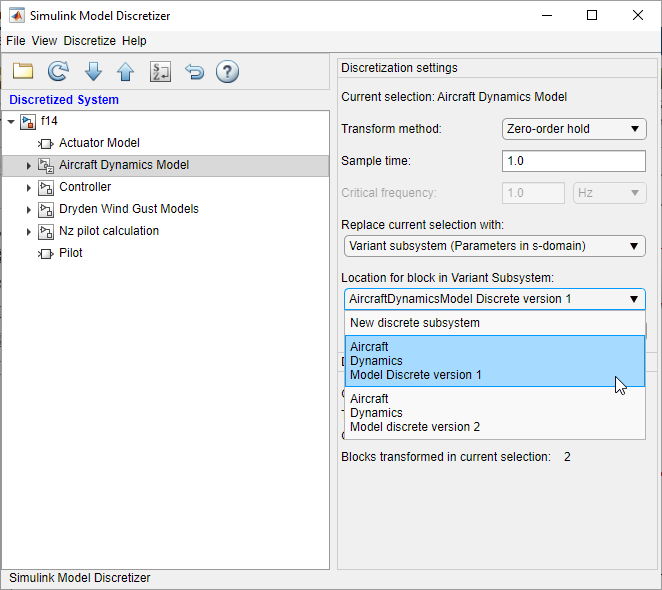The other blocks in this `f14` model have not been discretized.

The following figure shows the Aircraft Dynamics Model subsystem of the `f14` example model after discretization into a variant subsystem containing the original continuous model and two discretization candidates.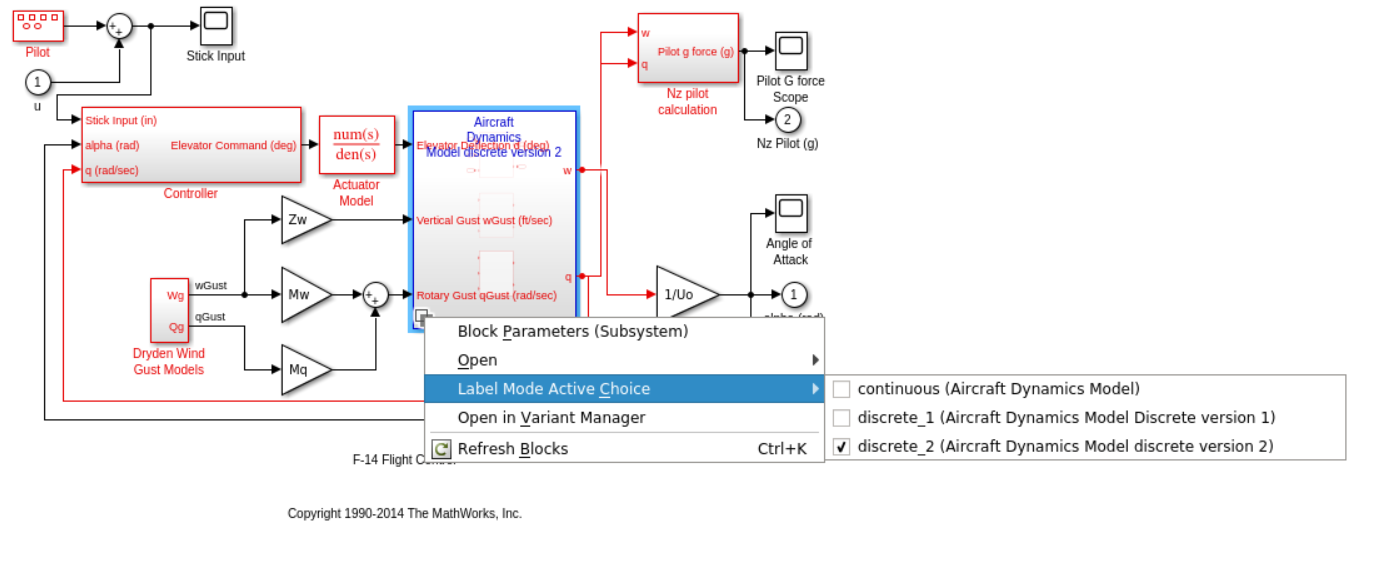The following figure shows the Aircraft Dynamics Model variant subsystem with the two discretization candidates.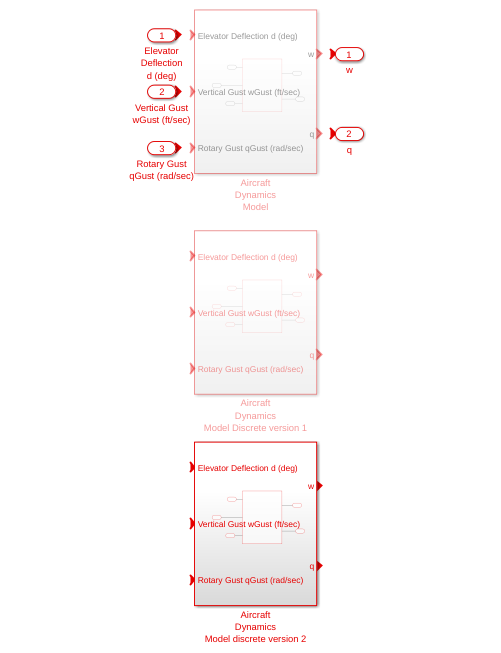#### Refreshing Model Discretizer View of the Model

To refresh the Model Discretizer tree view of the model when the model has been changed, click the button.

Alternatively, you can select View > Refresh.

### Discretize Blocks from the Simulink Model

You can replace continuous blocks in a Simulink software model with the equivalent blocks discretized in the s-domain using the Discretizing library.

The procedure below shows how to replace a continuous Transfer Fcn block in the Aircraft Dynamics Model subsystem of the `f14` model with a discretized Transfer Fcn block from the Discretizing Library. The block is discretized in the s-domain with a zero-order hold transform method and a two second sample time.

1. Open the `f14` model.

2. Open the Aircraft Dynamics Model subsystem in the `f14` model.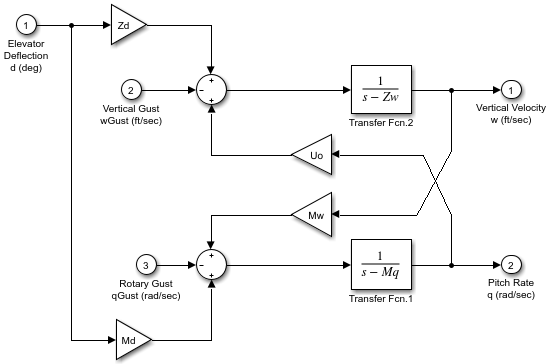3. Open the Discretizing library window.

Enter `discretizing` at the MATLAB command prompt.

The Library: discretizing window opens.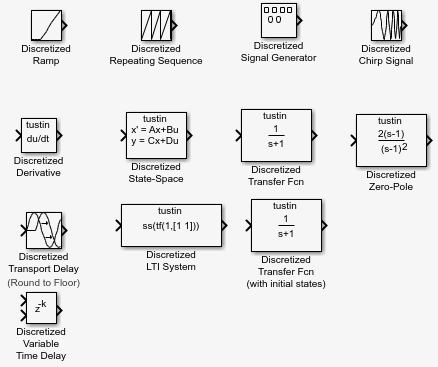This library contains s-domain discretized blocks.

4. Add the Discretized Transfer Fcn (with initial states) block to the f14/Aircraft Dynamics Model window.

1. Click the Discretized Transfer Fcn block in the Library: discretizing window.

2. Drag it into the f14/Aircraft Dynamics Model window.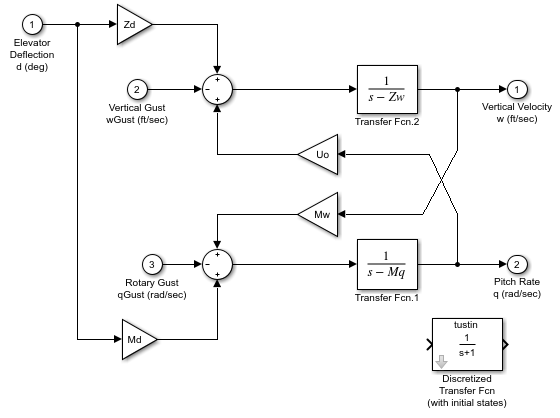5. Open the parameter dialog box for the Transfer Fcn.1 block.

Double-click the Transfer Fcn.1 block in the f14/Aircraft Dynamics Model window.

The Block Parameters: Transfer Fcn.1 dialog box opens.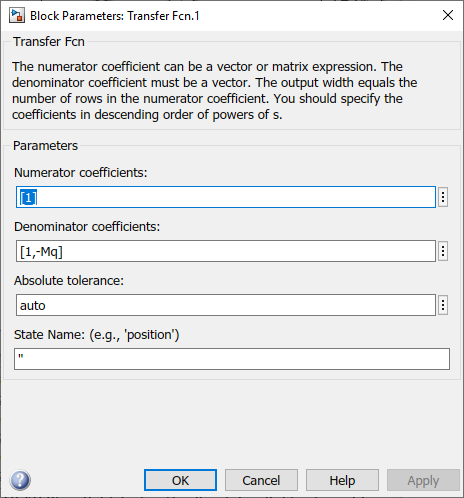6. Open the parameter dialog box for the Discretized Transfer Fcn block.

Double-click the Discretized Transfer Fcn block in the f14/Aircraft Dynamics Model window.

The Block Parameters: Discretized Transfer Fcn dialog box opens.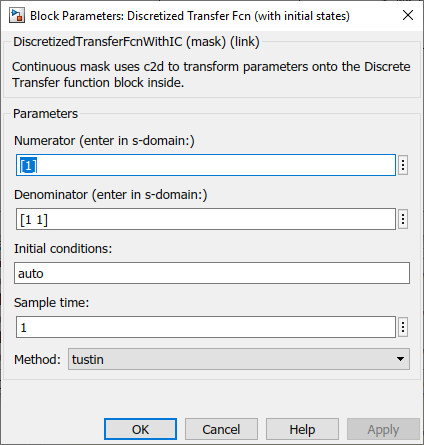Copy the parameter information from the Transfer Fcn.1 block dialog box to the Discretized Transfer Fcn block's dialog box.7. Enter `2` in the Sample time field.

8. Select `zoh` from the Method dropdown list.

The parameter dialog box for the Discretized Transfer Fcn now looks like this.9. Click .

The f14/Aircraft Dynamics Model window now looks like this.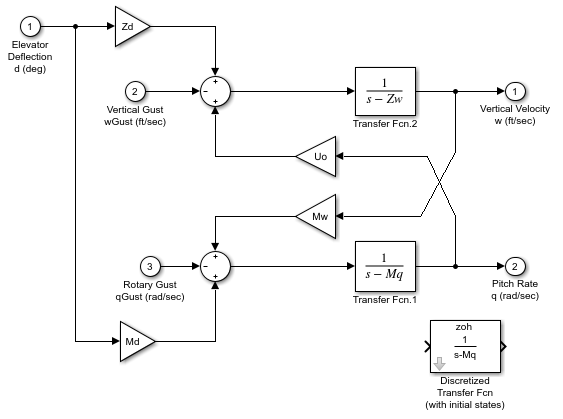10. Delete the original Transfer Fcn.1 block.

1. Click the Transfer Fcn.1 block.

2. Press the Delete key.

The f14/Aircraft Dynamics Model window now looks like this.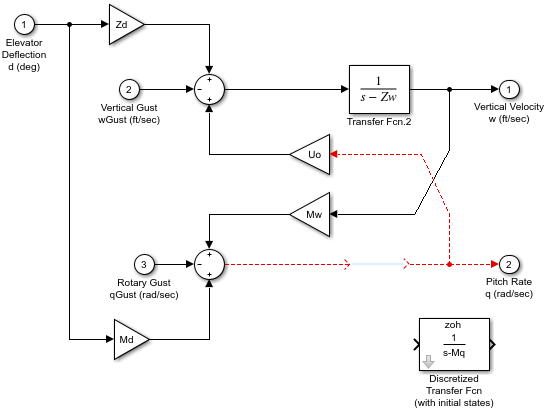11. Add the Discretized Transfer Fcn block to the model.

1. Click the Discretized Transfer Fcn block.

2. Drag the Discretized Transfer Fcn block into position to complete the model.

The f14/Aircraft Dynamics Model window now looks like this.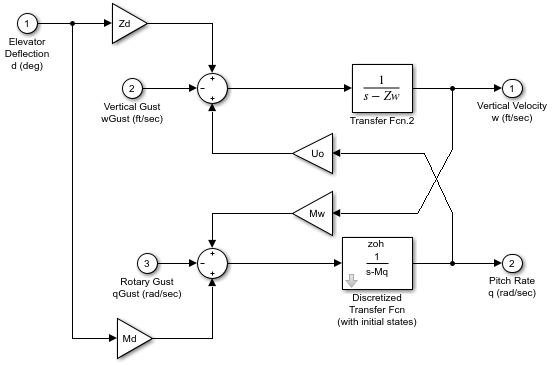### Discretize a Model with the sldiscmdl Function

Use the `sldiscmdl` function to discretize Simulink software models from the MATLAB Command Window. You can specify the transform method, the sample time, and the discretization method with the `sldiscmdl` function.

For example, the following command discretizes the `f14` model in the s-domain with a 1-second sample time using a zero-order hold transform method:

```sldiscmdl('f14',1.0,'zoh') ```